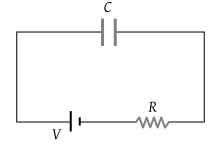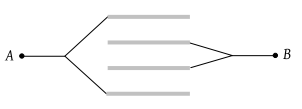If the distance between parallel plates of a capacitor is halved and dielectric constant is doubled then the capacitance

(1) Decreases two times

(2) Increases two times

(3) Increases four times

(4) Remain the same

Concept Questions :-

Capacitance
High Yielding Test Series + Question Bank - NEET 2020

Difficulty Level:

If there are n capacitors each of capacitance C in parallel connected to V volt source, then the energy stored is equal to

(1) CV

(2) $\frac{1}{2}nC{V}^{2}$

(3) CV2

(4) $\frac{1}{2n}C{V}^{2}$

Concept Questions :-

Energy stored in capacitor
High Yielding Test Series + Question Bank - NEET 2020

Difficulty Level:

A capacitor of capacitance 6 μF is charged upto 100 volt. The energy stored in the capacitor is

(1) 0.6 Joule

(2) 0.06 Joule

(3) 0.03 Joule

(4) 0.3 Joule

Concept Questions :-

Energy stored in capacitor
High Yielding Test Series + Question Bank - NEET 2020

Difficulty Level:

The unit of electric permittivity is

(1) Volt/m2

(2) Joule/coulomb

(4) Henry/m

Concept Questions :-

Capacitance
High Yielding Test Series + Question Bank - NEET 2020

Difficulty Level:

The work done in placing a charge of 8 × 10–18 coulomb on a condenser of capacity 100 micro-farad is

(1) 32× 10–32 Joule

(2) 16 × 10–32 Joule

(3) 3.1 × 10–26 Joule

(4) 4 × 10–10 Joule

Concept Questions :-

Energy stored in capacitor
High Yielding Test Series + Question Bank - NEET 2020

Difficulty Level:

A parallel plate capacitor of capacity C0 is charged to a potential V0

(i) The energy stored in the capacitor when the battery is disconnected and the separation is doubled E1

(ii) The energy stored in the capacitor when the charging battery is kept connected and the separation between the capacitor plates is doubled is E2. Then E1 / E2 value is

(1) 4

(2) 3/2

(3) 2

(4) 1/2

Concept Questions :-

Energy stored in capacitor
High Yielding Test Series + Question Bank - NEET 2020

Difficulty Level:

As in figure shown, if a capacitor C is charged by connecting it with resistance R, then energy  given by the battery will be(1) $\frac{1}{2}C{V}^{2}$

(2) More than $\frac{1}{2}C{V}^{2}$

(3) Less than $\frac{1}{2}C{V}^{2}$

(4) Zero

Concept Questions :-

RC circuit
High Yielding Test Series + Question Bank - NEET 2020

Difficulty Level:

Two identical capacitors are joined in parallel, charged to a potential V and then separated and then connected in series i.e. the positive plate of one is connected to negative of the other

(1) The charges on the free plates connected together are destroyed

(2) The charges on the free plates are enhanced

(3) The energy stored in the system increases

(4) The potential difference in the free plates becomes 2V

Concept Questions :-

Combination of capactiors
High Yielding Test Series + Question Bank - NEET 2020

Difficulty Level:

A parallel plate capacitor is made by stacking n equally spaced plates connected alternately. If the capacitance between any two plates is C then the resultant capacitance is

(1) C

(2) nC

(3) (n – 1)C

(4) (n + 1)C

Concept Questions :-

Combination of capactiors
High Yielding Test Series + Question Bank - NEET 2020

Difficulty Level:

Four plates of equal area A are separated by equal distances d and are arranged as shown in the figure. The equivalent capacity is(1) $\frac{2{\epsilon }_{0}A}{d}$

(2) $\frac{3{\epsilon }_{0}A}{d}$

(3) $\frac{4{\epsilon }_{0}A}{d}$

(4) $\frac{{\epsilon }_{0}A}{d}$

Concept Questions :-

Combination of capactiors